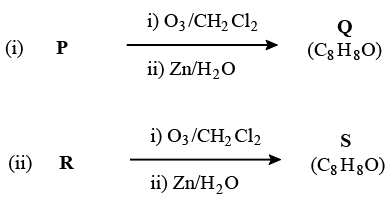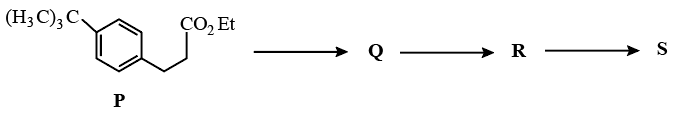Instructions

For the following questions answer them individually

Question 31

Question 32

# Compounds P and R upon ozonolysis produce Q and S, respectively. The molecular formula of Q and S is $$C_{8}H_{8}O$$. Q undergoes Cannizzaro reaction but not haloform reaction, whereas S undergoes haloform reaction but not Cannizzaro reaction.The option(s) with suitable combination of P and R, respectively, is(are)

Instructions

Upon heating $$KClO_{3}$$ in the presence of catalytic amount of $$MnO_{2}$$ a gas W is formed. Excess amount of W reacts with white phosphorus to give X. The reaction of X with pure $$HNO_{3}$$ gives Y and Z.

Question 33

Question 34

# Y and Z are, respectively

Instructions

The reaction of compound P with $$CH_{3}MgBr$$ (excess) in $$(C_{2}H_{5})_{2}O$$ followed by addition of $$H_{2}O$$ gives Q. The compound Q on treatment with $$H_{2}SO_{4}$$ at $$0^{0}_{C}$$ gives R. The reaction of R with $$CH_{3}COCl$$ in the presence of anhydrous $$AlCl_{3}$$ in $$CH_{2}Cl_{2}$$ followed by treatment with $$H_{2}O$$ produces compound S. [Et in compound P is ethyl group]Question 35

Question 36

# The reactions, Q to R and R to S, are

Instructions

For the following questions answer them individually

Question 37

Question 38

Question 39

Question 40

OR